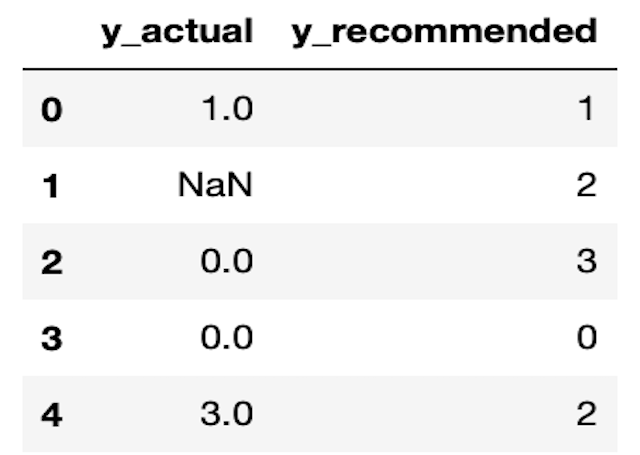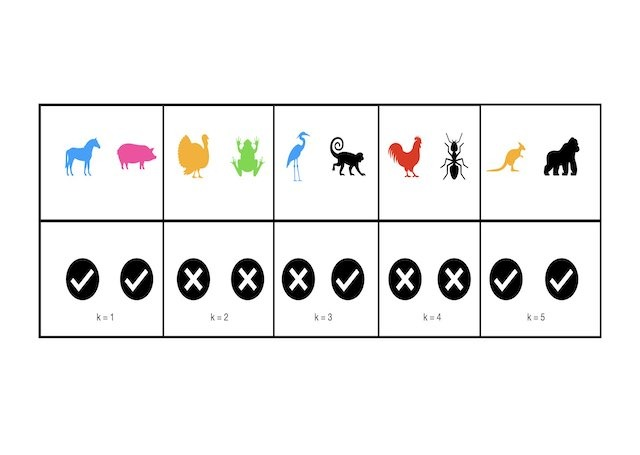# Classification

This category groups articles on the subject of classification. Each post focuses on either a specific classification algorithm, or a tool used when tackling a classification problem. The emphasis here is on understanding these models and techniques at a technical level. Here you will learn to build classification models in Python from scratch.## Can Decision Trees Handle Categorical Features?

Can Decision Trees Handle Categorical Features? Yes, Decision Trees handle categorical features naturally. Often these features are treated by first one-hot-encoding (OHE) in a preprocessing step. However, it is straightforward to extend the CART algorithm to make use of categorical features without such preprocessing. In this post, I will implement classification and regression Decision Trees capable …## Can Decision Trees Handle Missing Values?

Can Decision Trees Handle Missing Values? Yes, Decision Trees handle missing values naturally. It is straightforward to extend the CART algorithm to support the handling of missing values. However, attention needs to be made regarding how the algorithm is implemented in code. In this post, I will implement classification and regression Decision Trees capable of dealing …## 3 Methods to Tune Hyperparameters in Decision Trees

3 Methods to Tune Hyperparameters in Decision Trees We can tune hyperparameters in Decision Trees by comparing models trained with different parameter configurations, on the same data. An optimal model can then be selected from the various different attempts, using any relevant metrics. There are several different techniques for accomplishing this task. Three of the …## How to Measure Information Gain in Decision Trees

How to Measure Information Gain in Decision Trees For classification problems, information gain in Decision Trees is measured using the Shannon Entropy. The amount of entropy can be calculated for any given node in the tree, along with its two child nodes. The difference between the amount of entropy in the parent node, and the …## [email protected] and [email protected] Made Easy with 1 Python Example

[email protected] and [email protected] Made Easy with 1 Python Example What are [email protected] and [email protected] ? [email protected] and [email protected] are metrics used to evaluate a recommender model. These quantities attempt to measure how effective a recommender is at providing relevant suggestions to users. The typical workflow of a recommender involves a series of suggestions that will be …## Implement the KNN Algorithm in Python from Scratch

Implement the KNN Algorithm in Python from Scratch In this post, we will cover the K Nearest Neighbours algorithm: how it works and how it can be used. We will work through implementing this algorithm in Python from scratch, and verify that our model works as expected. What is the KNN Algorithm? K Nearest Neighbours …## A Complete Introduction to Cross Validation in Machine Learning

A Complete Introduction to Cross Validation in Machine Learning This post will discuss various Cross Validation techniques. Cross Validation is a testing methodology used to quantify how well a predictive machine learning model performs. Simple illustrative examples will be used, along with coding examples in Python. What is Cross Validation? A natural question to ask, when …